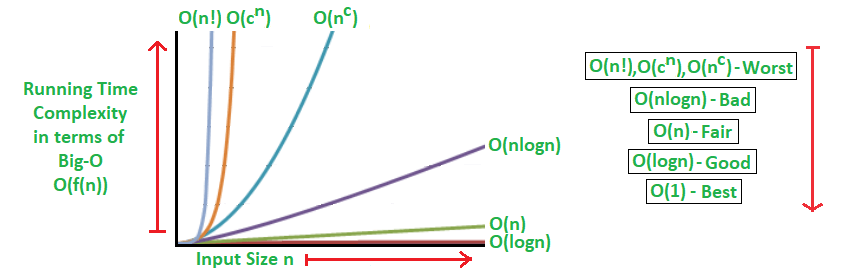Posted on

## Big Oh(O) Big Theta(Θ) Big Omega(Ω)## Analysis of Algorithms

Big O notation is a mathematical notation that describes the limiting behavior of a function when the argument tends towards a particular value or infinity. It is a member of a family of notations invented by Paul Bachmann, Edmund Landau, and others, collectively called Bachmann–Landau notation or asymptotic notation.Big Oh Running Time Complexity

Two simple concepts separate properties of an algorithm itself from properties of a particular computer, operating system, programming language, and compiler used for its implementation. The concepts, briefly outlined earlier, are as follows:

The input data size, or the number n of individual data items in a single data instance to be processed when solving a given problem. Obviously, how to measure the data size depends on the problem: n means the number of items to sort (in sorting applications), number of nodes (vertices) or arcs (edges) in graph algorithms, number of picture elements (pixels) in image processing, length of a character string in text processing, and so on.

The number of elementary operations taken by a particular algorithm, or its running time. We assume it is a function f(n) of the input data size n. The function depends on the elementary operations chosen to build the algorithm.

Algorithms are analyzed under the following assumption: if the running time of an algorithm as a function of n differs only by a constant factor from the running time for another algorithm, then the two algorithms have essentially the same time complexity. Functions that measure running time, T(n), have nonnegative values
because time is nonnegative, T(n) ≥ 0. The integer argument n (data size) is also nonnegative.

### Definition 1 (Big Oh)Big Oh Rank Meaning

Let f(n) and g(n) be nonnegative-valued functions defined on nonnegative integers n. Then g(n)is O(f(n)) (read “g(n)is Big Oh of f(n)”) iff there exists a positive real constant c and a positive integer n0 such that g(n) ≤ c f(n) for all n > n0.

Note. We use the notation “iff ” as an abbreviation of “if and only if”.

Continue reading Big Oh(O) Big Theta(Θ) Big Omega(Ω)